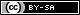# Dev Blog

 ./dev Original heme by orderedlist (CC-BY-SA) Where applicable, all content is licensed under a CC-BY-SA.# Amdahl's Law

• $T_s$ - Time for a serial task
• $p$ - portion of a task that can be parallelized ($p \in [0,1]$)
• $n$ - number of processes that can be used to parallelize
• $T_{p,n}$ - Time for a parallel task with $p$ and $n$
• $F_{p,n} = \frac{T_s}{T_{p,n}}$ - Speedup factor for $p$ and $n$

$$T_{p,n} = T_s (1-p) + \frac{T_s p}{n} \\ \to \frac{T_{p,n}}{T_s} = 1 - p + \frac{p}{n} \\ \to \frac{T_s}{T_{p,n}} = \frac{1}{ 1 - p + \frac{p}{n} } \\ \to F_{p,n} = \frac{1}{ 1 - p + \frac{p}{n} }$$

For a completely serial task ($p=0$):

$$F_{p,n} = \frac{1}{ 1 - 0 + \frac{0}{n} } = 1$$

For a completely parallel task ($p=1$):

$$F_{p,n} = \frac{1}{ 1 - 1 + \frac{1}{n} } = \frac{1}{ \frac{1}{n} } = n$$

When $n >> 1$:

$$F_{p,n} = \frac{1}{ 1 - p + \frac{p}{n} } = \frac{n}{ n - n p + p } \\ \approx \frac{n}{n -n p} = \frac{1}{1-p}$$